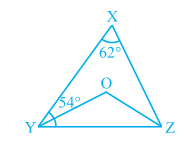Q

# In Fig. 6.40, ∠ X = 62°, ∠ XYZ = 54°. If YO and ZO are the bisectors of ∠ XYZ and ∠ XZY respectively of ∆ XYZ, find ∠ OZY and ∠ YOZ.

2. In Fig. 6.40, X = 62°, XYZ = 54°. If YO and ZO are the bisectors of XYZ and XZY respectively of XYZ, find OZY and YOZ.ViewsWe have
X = XYZ =
YO and ZO bisects the XYZ and XZY
Now, In XYZ, by using angle sum property
XYZ + YZX + ZXY =

So, YZX =
YZX =

and, OYZ =  also, OZY =

Now, in OYZ
Y + O + Z =   [Y =  and Z = ]
So, YOZ =

Exams
Articles
Questions